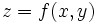# Bernstein minimal surface theorem

## Statement

Any minimal surface embedded in$\R^3$ with a Cartesian equation of the form:$z = f(x,y)$

where$f$ is defined for all real$x,y$ and has continuous partial derivatives in both$x$ and$y$, must be a plane.